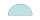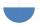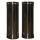# Perimeter + real numbers - math problems

#### Number of problems found: 12

• DescribedCalculate perimeter of the circle described by a triangle with sides 478, 255, 352.
• Quarter circularThe wire that is hooked around the perimeter of quarter-circular arc has length 3π+12. Determine the radius of circle arc.
• Rectangle SSPerimeter of a rectangle is 268 cm and its diagonal is 99.3 cm. Determine the dimensions of the rectangle.
• AnglesDetermine the interior angles of a rhombus with area 319.1 cm2 and perimeter 72 cm.
• GardenHow many steps of 76 cm circumvent square garden with area 1.8 ha?
• EstateSemicircle estate must be fence. The straight section has 26 meters long fence. How many meters of fence should buy?
• Wire DWire length 1 m is bent so that it forms a semicircle circuit (including the diameter). Determine the radius of the semicircle.
• Rhombus ABCDRhombus ABCD, |AC| = 90 cm, |BD| = 49 cm. Calculate the perimeter of the rhombus ABCD.
• CylindersThe area of the side of two cylinders is the same rectangle of 33 mm × 18 mm. Which cylinder has a larger volume and by how much?
• Short cutImagine that you are going to a friend. That path has a length 120 meters. Then turn doprava and go other 630 meters and you are at a friend's. The question is how much the journey will be shorter if you go direct across the field?
• TinsmithTinsmith construct chimney pipe 145 cm long and 15 cm wide. What are the dimensions of the sheet will have to prepare for the construction of a pipe when the plate overlap at the joint add \$x cm width of the plate?
• GardenThe area of a square garden is 6/4 of triangle garden with sides 56 m, 35 m, and 35 m. How many meters of fencing need to fence a square garden?

We apologize, but in this category are not a lot of examples.
Do you have an interesting mathematical word problem that you can't solve it? Submit a math problem, and we can try to solve it.

We will send a solution to your e-mail address. Solved examples are also published here. Please enter the e-mail correctly and check whether you don't have a full mailbox.

Please do not submit problems from current active competitions such as Mathematical Olympiad, correspondence seminars etc...

Perimeter - math problems. Real numbers - math problems.